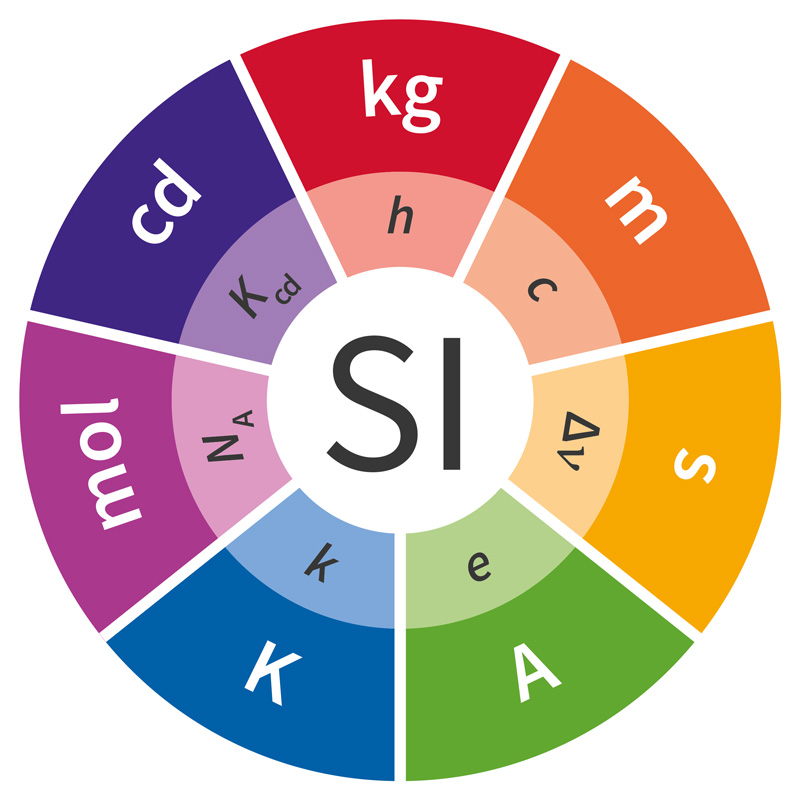# The revised International System of Units (SI) explained

In 1948 the CGPM ratified this definition of the ampere: "The ampere is that constant current which, if maintained in two straight parallel conductors of infinite length, of negligible circular cross-section, and placed 1 metre apart in vacuum, would produce between these conductors a force equal to 2 × 10−7 MKS unit of force [newton] per metre of length". With the same definition, the ampere was then chosen in 1954 as one of the base units of a practical system of units, that eventually became the SI in 1960.

Since 1960, new units for electric quantities have been introduced (for example the siemens, i.e., reciprocal ohm, in 1971) and better strategies of realization of the definition of the units have been adopted (for example the Josephson effect for the realization of the volt and the quantum Hall effect for the realization of the ohm, in 1988), but the definition of the ampere has remained unchanged. Indeed, until 2019, the definition of the ampere in the SI [as reflected in the Electropedia (IEV 112-02-07:2010-01)] was substantially that given in 1948: "SI unit of electric current, equal to the direct current which, if maintained constant in two straight parallel conductors of infinite length, of circular cross-section with negligible area, and placed 1 metre apart in vacuum, would produce between these conductors a force per length equal to 2 × 10−7 N/m".

Such a definition has been the subject of criticism on the grounds that any realization can only be an approximation of what the definition says: no two conductors are perfectly straight, parallel, of infinite length, and so on. Someone even came to the most radical conclusion that, given its definition, the ampere is only an ideal entity, which does not exist as the intensity of an actual electric current!The base units in the revised SI together
with their defining constants

With the twofold aim of avoiding these criticisms and of improving the stability of the SI by defining its base units in terms of constant quantities, several years of research were devoted, and these have led finally to a new way to conceive the SI that came into effect in 2019. In short, the so-called "revised SI" is based on establishing the numerical values of some constant quantities, from which the definitions of the base units are deduced.

In particular, the charge of the electron e, that the current physical theories consider as a fundamental constant of nature, is set to 1,602 176 634 × 10−19 C (coulomb). From this, the ampere is then defined by simply taking this information in inverse form (and under the assumption that the second is in turn defined): "The ampere, symbol A, is the SI unit of electric current. It is defined by taking the fixed numerical value of the elementary charge e to be 1,602 176 634 × 10−19 when expressed in the unit C, which is equal to A s, where the second is defined in terms of ΔvCs."

This new style of definition has been called the “explicit constants”, or “constants first”, approach because the definitions of the units are deducted from the setting of the numerical values of the constants, and not vice versa as before. While the change is radical from the point of view of the structure of the definitions, it should be noted that the numerical values of the defining constants have been carefully chosen so as to guarantee that the new definitions identify the same quantities as before: in particular, the "1948 ampere" and the "2019 ampere", although defined in a completely different way, are substantially the same intensity of electric current, of course within the limits of the currently available knowledge.